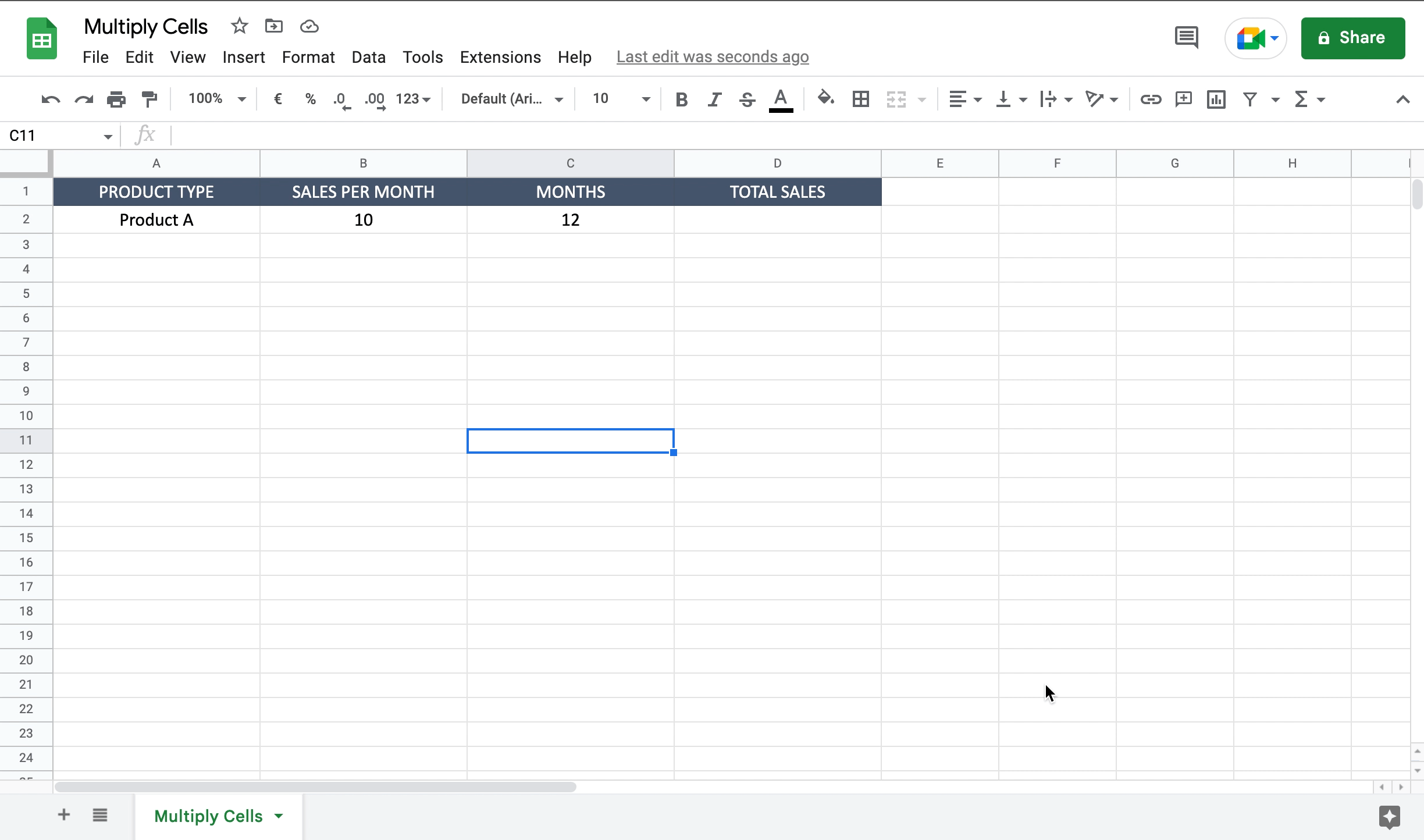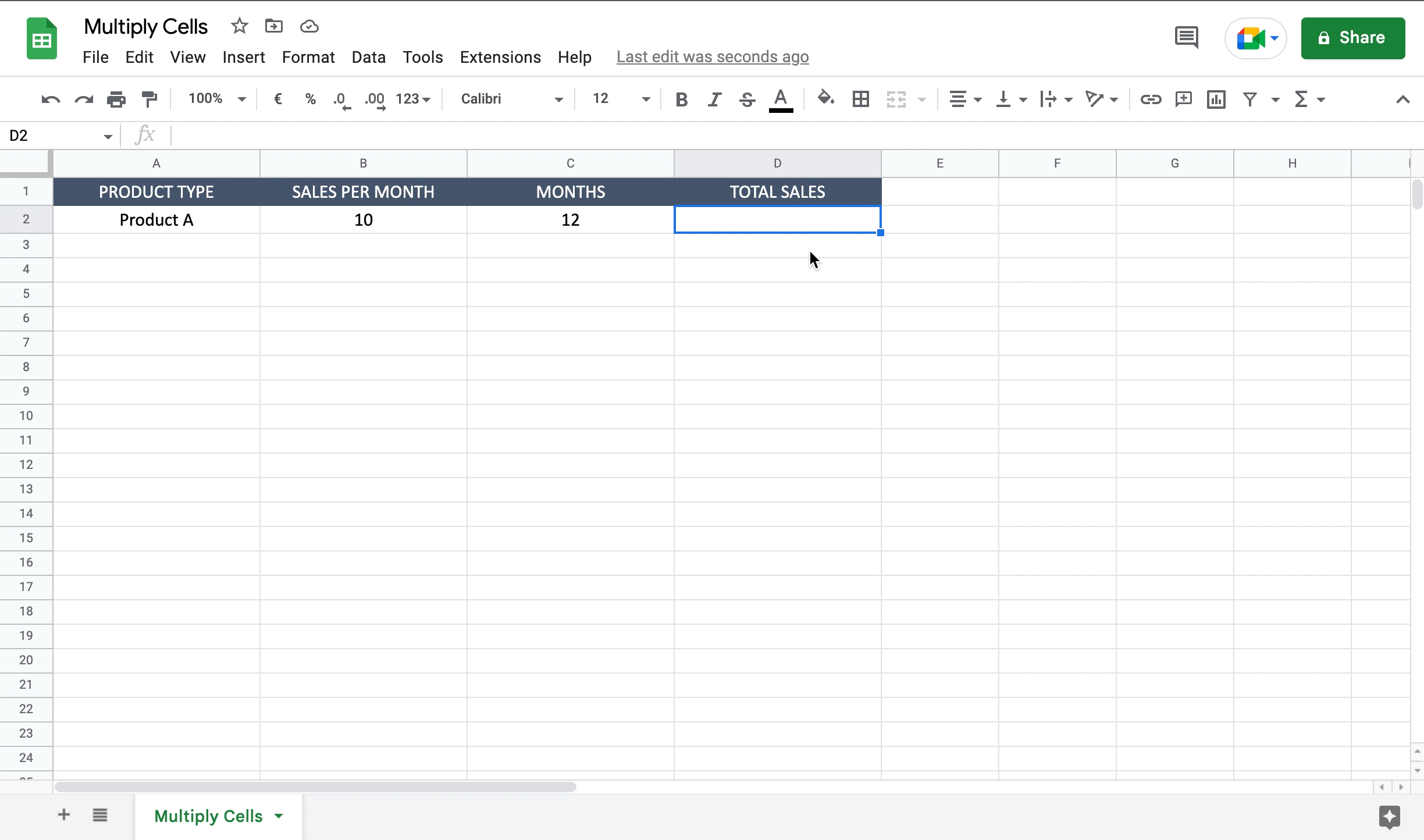# How to multiply cells in Google SheetsLet’s assume you want to calculate how many items of a certain product you sold during a year. You have two columns, one with the number of products sold each month and one with the number of months. By multiplying the two cells you’ll obtain the number of yearly sales. To multiply cells in Google Sheets proceed as follows.

### Step 1 – Select the cell where to insert the multiplication– Select the cell by clicking on it or with the keyboard arrows. A good choice can be to select the cell near the two you want to multiply, in order to have the result as close as possible.

### Step 2 – Multiply the two cells– Inside the selected cell write “=” to let the tool understand you want to insert a function;
– Select the first cell you want to multiply adding it to the function;
– Write “*” as multiplication operator;
– Select the second cell you want to multiply adding it to the function;
– Press enter to confirm the function.